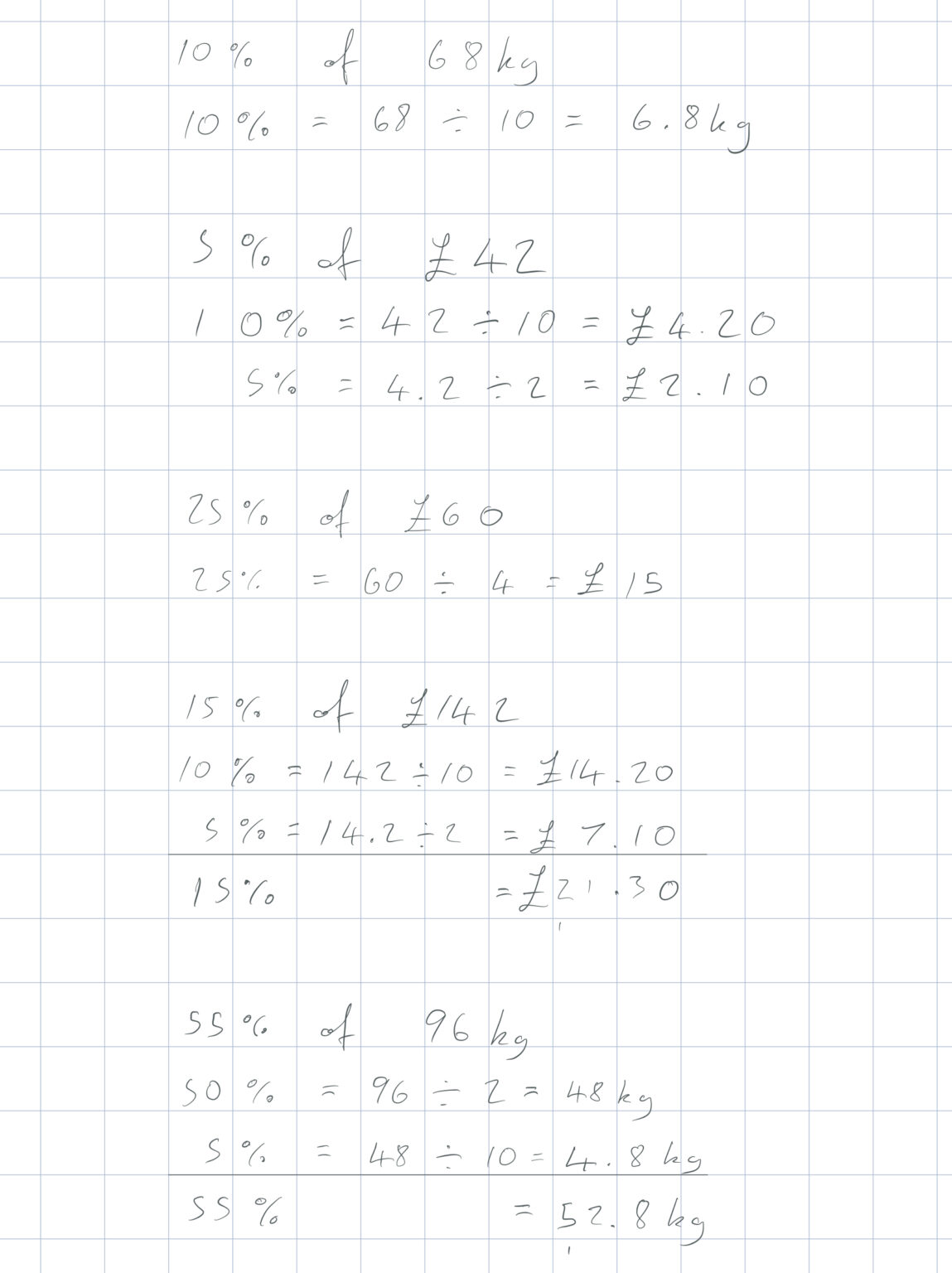There are several percentages we can find easily without a calculator:

10% (divide 100% by 10), 50% (divide 100% by 2), 25% (divide 100% by 4), 33 ⅓% (divide 100% by 3).

We can find any other percentage by using the above percentages. The most useful is 10%: We can find 5% by dividing 10% by 2. We can find 1% by dividing 10% by 10. We can find 30% by multiply 10% by 3, etc.